# Tubing Length Change due to Thermal Load

Difference in temperature causes steel to contract or expand. If tubing is free to move, length of the tubing will be either longer or shorter due to thermal expansion. On the other hand, if the tubing is not free to move, there will be a change in axial force due to the temperature effect.

Figure 1 illustrates an increase in length due to heat and Figure 2 demonstrates a decrease in length because of cooling.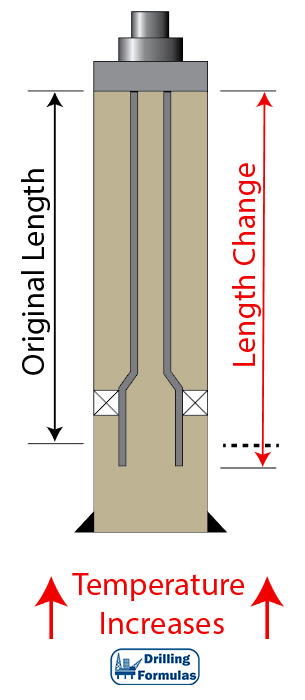Figure 1 – Tubing Lengthen by Temperature Increase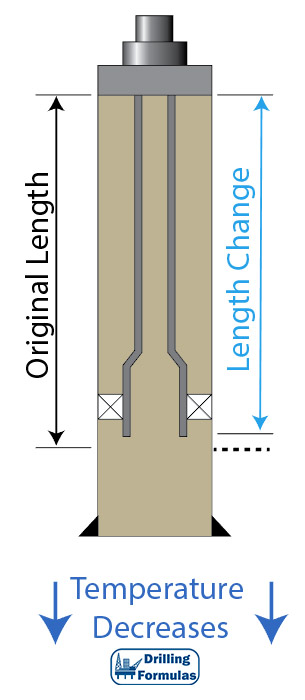Figure 2 – Tubing Shorten by Temperature Decrease

Magnitude of contraction or expansion force is dependent on expansion coefficient of steel (Ct).

If tubing is anchored, the force generated by temperature change is calculated by the following equation.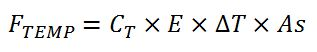Where;

FTEMP = Force generated by change in temperature (inch)

CT = Thermal expansion coefficient (1/F)

E = Young’s Modulus of material (psi)

ΔT = Average temperature change from the initial condition to the final condition

As = Cross sectional area of tubular (inch2)

Average Temperature = (Surface Temperature + Bottom Hole Temperature) ÷2 (F)

If tubing is free to move, the force generated by temperature change is calculated by the following equation.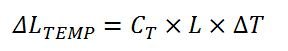Where;

ΔLTEMP = Length change due to thermal effect (inch)

CT = Thermal expansion coefficient (1/F)

L = Length of tubing (inch)

ΔT = Average temperature change from the initial condition to the final condition (F)

Average Temperature = (Surface Temperature + Bottom Hole Temperature) ÷2 (F)

Thermal changes can happen during the life of the well. While producing, heat from the reservoir will expand the length of the tubing, whereas an injection operation will contract tubular due to cooling down the temperature. Figure 3 shows the difference in temperature gradient while the well is on production or injection. Therefore, it is important to understand how thermal will affect changes in length or force in the tubular.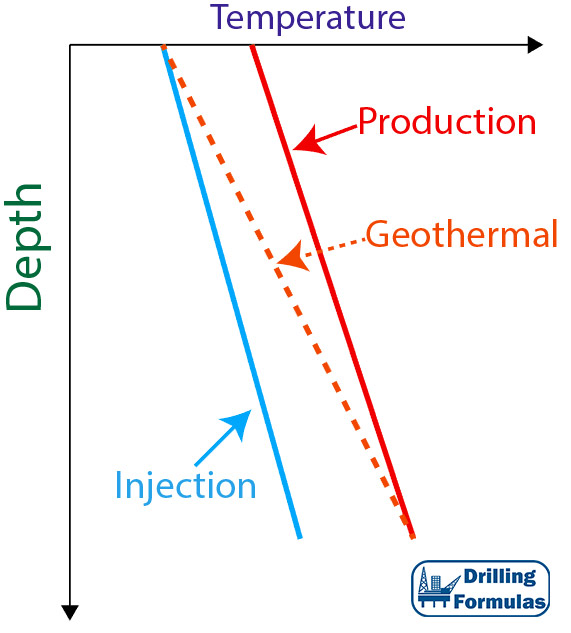Figure 3 – Temperature Gradient During Life of The Well

ExampleThis example will demonstrate how to calculate a length change due to thermal effect.

Tubing is free to move.

Packer setting depth is 10,000 ft.

CT = 6.9×10-6 (1/F)

At the initial condition

Surface temperature (F) = 60F

Bottom hole temperature (F) = 150 F

At the final condition

Surface temperature (F) = 90F

Bottom hole temperature (F) = 150 F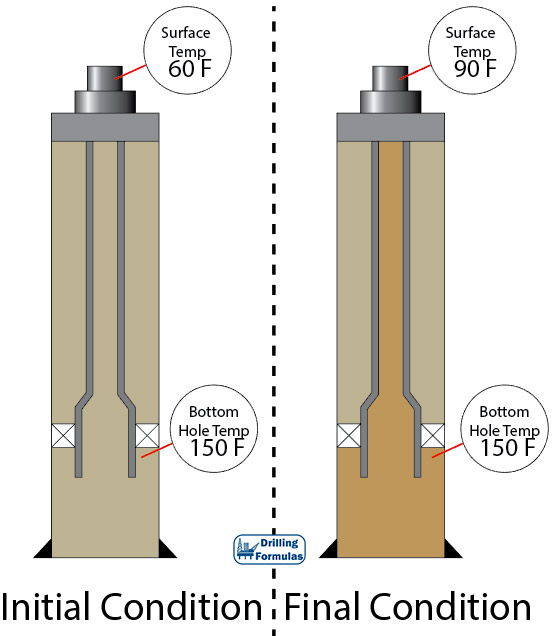Figure 4 – The diagram of the well at initial and final condition

Solution

Length of tubing (inch) 12 × 10,000 = 120,000 inch

Average Temperature at Initial Condition = (60 + 150) ÷2 = 105 F

Average Temperature at Final Condition = (90 + 150) ÷2 = 120 F

ΔT = 120 – 105 = 15 F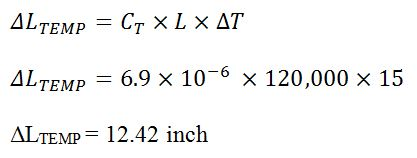ΔLTEMP = 12.42 inch

Conclusion

Length of tubular will increase by 12.42 inches due to thermal affect based on the given information.

References

Jonathan Bellarby, 2009. Well Completion Design, Volume 56 (Developments in Petroleum Science). 1 Edition. Elsevier Science.

Wan Renpu, 2011. Advanced Well Completion Engineering, Third Edition. 3 Edition. Gulf Professional Publishing.

Ted G. Byrom, 2014. Casing and Liners for Drilling and Completion, Second Edition: Design and Application (Gulf Drilling Guides). 2 Edition. Gulf Professional Publishing.

Lubinski, A., & Althouse, W. S. (1962, June 1). Helical Buckling of Tubing Sealed in Packers. Society of Petroleum Engineers. doi:10.2118/178-PA

Share the joy
Tagged , , , , , . Bookmark the permalink.Working in the oil field and loving to share knowledge.

### 5 Responses to Tubing Length Change due to Thermal Load

1.Moh khalil says:

Thank you for a good explaination.
Only one thing you forget is the equation in case free movement pipe or anchored pipe.

•DrillingFormulas.Com says:

Moh,

This issue is fixed.
Thanks for information.

Regards,
Shyne

2.moh. khalil says:

Thank you Shyne. Just one point to be considered for anchored tubing. The equation for the force should be without “E” (Young Modulus), Thanks again for a good Website.

•DrillingFormulas.Com says:

I will add more details later.

3.reza says:

Dear sir,

Thanks a lot for useful articles.